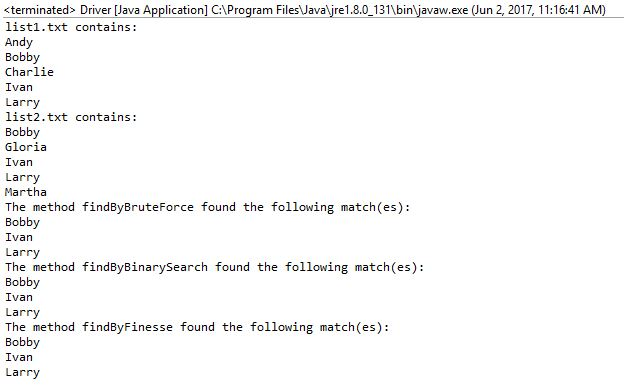# Question & Answer: Goal Build three search algorithms to find matching names in two different lists using Java. ____ Problem…..

Goal

Build three search algorithms to find matching names in two different lists using Java.

Don't use plagiarized sources. Get Your Custom Essay on
Question & Answer: Goal Build three search algorithms to find matching names in two different lists using Java. ____ Problem…..
GET AN ESSAY WRITTEN FOR YOU FROM AS LOW AS \$13/PAGE

____

Problem

1. Read two input files, each containing a sorted list of first names. Assume the input

files will be in the working directory and will be named list1.txt and list2.txt.

2. Find the matching names using three different algorithms. The first list could be

considered the reference list, and the second list would contain the list of names to be

found.

(a) The first search method, named findByBruteForce should use a linear search of

the target list. The inputs to this function will be the two lists.

(b) The second search method, named findByBinarySearch should implement a binary

search algorithm. The inputs to this function will be the two lists.

(c) The third search method, named findByFinesse should implement the tokenized

search discussed in class and summarized below.

Start two markers, one for each list, at the beginning of each list.

Repeat the process outlined below

Compare the two marked items

If both are equal print the name and advance both markers one element.

else since they’re not equal advance the marker that comes first, alphabetically.

____

Testing

There are three files supplied with the assignment:

1. list1.txt which contains the list of five names.

2. list2.txt also contains a list of five names.

3. expectedOutput.txt which is a redirection of the output. This is to be used as the

baseline for running the diff command to confirm the output results and formatting.

(See the Sample Output below for an example of how diff will be used.)

____

Sample output

java Lab01

list1.txt contains:

list2.txt contains:

The method findByBruteForce found the following match(es):

The method findByBinarySearch found the following match(es):

The method findByFinesse found the following match(es):

java Lab01 >myOutput.txt

diff myOutput.txt expectedOutput.txt

Note The diff command will report any differences between the input files. No output means there is no differences between the input files.

import java.io.IOException;

public class SearchMethods {

String[] list1;
String[] list2;

public SearchMethods(String[] list1, String[] list2) {
super();
this.list1 = list1;
this.list2 = list2;

}

//brute force
public void findByBruteForce(){
System.out.println(“The method findByBruteForce found the following match(es):”);
for(String s:list1){

for(String t:list2){
if(s.equals(t)){

System.out.println(s);;
break;
}
}
}

}

//binary search
public void findByBinarySearch() throws IOException{

System.out.println(“The method findByBinarySearch found the following match(es):”);
for(String s:list1){

int f = bsearch(s,list2,0,list2.length);
if(f>=0){
System.out.println(s);
}

}

}

private int bsearch(String word, String [] words, int a, int b) {
if(b <= a)
return -1;

if(b – a == 1)
return words[a].equals(word) ? a : -1;

int p = (a + b)/2;
if(word.compareTo(words[p]) < 0) {
return bsearch(word, words, 0, p);
} else if(word.compareTo(words[p]) > 0) {
return bsearch(word, words, p, b);
}

return p;
}

public void findByFinesse() throws IOException{

int a = 0;
int b = 0;
System.out.println(“The method findByFinesse found the following match(es):”);
while(a<5 && b<5){
int c = list1[a].compareTo(list2[b]);
if(c==0){
System.out.println(list1[a]);
a++;
b++;
}else{
if(c<0) a++;
else b++;
}
}

}

}

import java.io.FileInputStream;

public class Driver {

public static void main(String[] args) {

//define the file size
final int sz = 5;

String[] f1 = new String[sz];
String[] f2 = new String[sz];

try{
FileInputStream file = new FileInputStream(“file1.txt”);

int i = 0;
String strline;
System.out.println(“list1.txt contains:”);
while ((strline = br.readLine()) != null && i<5) {
f1[i] = strline;
i++;
System.out.println(strline);
}

file = new FileInputStream(“file2.txt”);

i = 0;
System.out.println(“list2.txt contains:”);
while ((strline = br.readLine()) != null && i<5) {
f2[i] = strline;
i++;
System.out.println(strline);
}

br.close();

SearchMethods sm = new SearchMethods(f1, f2);

//Invoke whichever method is required
sm.findByBruteForce();
sm.findByBinarySearch();
sm.findByFinesse();
}
catch(Exception e){
e.printStackTrace();
}
}

}

//——————–OUTPUT——————————